# CLASS 8 MATHS CHAPTER 12 EXPONENTS AND POWERS

### Exercise 12.1

Question 1.

Evaluate:

(i)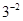(ii)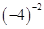(iii)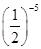Solution :

(i)= 1/3²

= 1/9

(ii)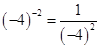= 1/16

(iii)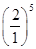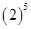= 32

Question 2.

Simplify and express the result in power notation with positive exponent:

(i)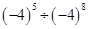(ii)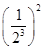(iii)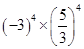(iv)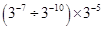(v)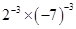Solution :

(i)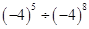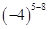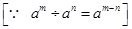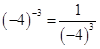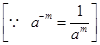(ii)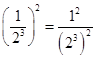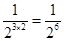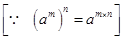(iii)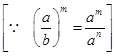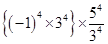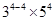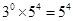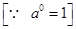(iv)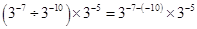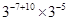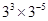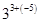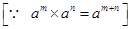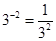Question 3.

Find the value of:

Solution :

Question 4.

Evaluate:

Solution :

= 1/10 × 1/6 = 1/60

Question 5.
Find the value of m for which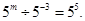Solution :

Comparing exponents both sides, we get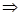m+3 = 5m = 5 – 3m = 2

Question 6.
Evaluate:

Question 7.
Simplify:

### NCERT Solutions for Class 8 Maths Exercise 12.2

Question 1.

Express the following numbers in standard form:

(i) 0.0000000000085

(ii) 0.00000000000942

(iii) 6020000000000000

(iv) 0.00000000837

(v) 31860000000

Solution :

(i) 0.0000000000085

= 0.0000000000085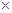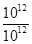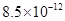(ii) 0.00000000000942

= 0.00000000000942=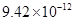(iii) 6020000000000000

= 6020000000000000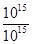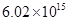(iv) 0.00000000837

= 0.00000000837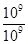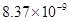(v) 31860000000 = 31860000000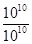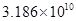Question 2.

Express the following numbers in usual form :

Solution :

Question 3.

Express the number appearing in the following statements in standard form :

(i)1 micron is equal to 1/ 1000000 m.

(ii) Charge of an electron is 0.000,000,000,000,000,000,16 coulomb.

(iii) Size of a bacteria is 0.0000005 m.

(iv) Size of a plant cell is

0.00001275 m.

(v) Thickness if a thick paper is 0.07 mm.

Solution :

(i) 1 micron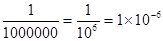m

(ii)Charge of an electron is

0.00000000000000000016 coulombs.

= 0.00000000000000000016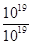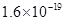coulomb

(iii) Size of bacteria = 0.0000005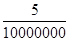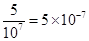m

(iv) Size of a plant cell is 0.00001275 m

= 0.00001275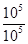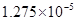m

(v) Thickness of a thick paper = 0.07 mm

= 7/100mm =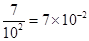mm

Question 4.

In a stack there are 5 books each of thickness 20 mm and 5 paper sheets each of thickness 0.016 mm. What is the total thickness of the stack?

Solution :

Thickness of one book = 20 mm

Thickness of 5 books = 20 × 5 = 100 mm

Thickness of one paper = 0.016 mm

Thickness of 5 papers = 0.016 × 5

= 0.08 mm

Total thickness of a stack = 100 + 0.08

=100.08 mm

= 100.08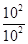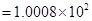mm

Get 30% off your first purchase!

X
error: Content is protected !!
Scroll to Top